# Conditional Probability

Suppose you and a friend wish to play a game that involves choosing a single card from a well-shuffled deck. Your friend deals you one card, face down, from the deck and offers you the following deal: if the card is a king, he will pay you $5, otherwise, you pay him$1. Should you play the game?

You reason in the following manner. Since there are four kings in the deck, the probability of obtaining a king is 4/52 or 1/13. And, the probability of not obtaining a king is 12/13. This implies that the ratio of your winning to losing is 1 to 12, while the payoff ratio is only $1 to$5. Therefore, you determine that you should not play.

Now consider the following scenario. While your friend was dealing the card, you happened to get a glance of it and noticed that the card was a face card. Should you, now, play the game?

Since there are 12 face cards in the deck, the total elements in the sample space are no longer 52, but just 12. This means the chance of obtaining a king is 4/12 or 1/3. So your chance of winning is 1/3 and of losing 2/3. This makes your winning to losing ratio 1 to 2 which fares much better with the payoff ratio of $1 to$5. This time, you determine that you should play.

In the second part of the above example, we were finding the probability of obtaining a king knowing that a face card had shown. This is an example of conditional probability. Whenever we are finding the probability of an event E under the condition that another event F has happened, we are finding conditional probability.

The symbol P(EF) denotes the problem of finding the probability of E given that F has occurred. We read P(EF) as “the probability of E, given F.”

Example 6.4.1

A family has three children. Find the conditional probability of having two boys and a girl given that the first born is a boy.
Solution
Let event E be that the family has two boys and a girl, and F the event that the first born is a boy. First, we list the sample space for a family of three children as follows.
S = {BBB , BBG , BGB , BGG , GBB , GBG , GGB , GGG}
Since we know that the first born is a boy, our possibilities narrow down to four outcomes, BBB , BBG , BGB , and BGG.
Among the four, BBG and BGB represent two boys and a girl.
Therefore P(E | F) = 2/4 or 1/2.

Conditional probability formula

For two events E and F, the probability of E given F is:

P(E | F) =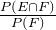Example 6.4.2

A single die is rolled. Use the above formula to find the conditional probability of obtaining an even number given that a number greater than three has shown.
Solution
Let E be the event that an even number shows, and F be the event that a number greater than three shows. We want P(E | F).
E = {2, 4, 6} and F = {4, 5, 6}. Which implies,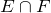= {4, 6}
Therefore, P(F) = 3/6, and P() = 2/6
P(E | F) =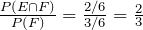.

Example 6.4.3

The following table shows the distribution by gender of students at a university who take public transportation and the ones who drive to school.
 Male (M) Female (F) Total Public Transportation (P) 8 13 21 Drive (D) 39 40 79 Total 47 53 100

The events M, F, P, and D are self explanatory. Find the following probabilities:

a. P(D | M)
b. P(F | D)
c. P(M | P)
Solution
We use the conditional probability formula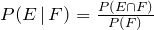.
a.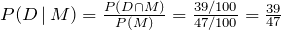.
b.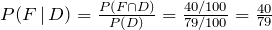.
c.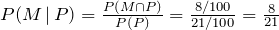.

Example 6.4.4

Given P(E) = 0.5, P(F) = 0.7, and P() = 0.3. Find the following.
a. P(E | F)
b. P(F | E).
Solution
We use the conditional probability formula.
a.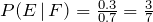.
b.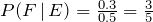.

Example 6.4.5

Given two mutually exclusive events E and F such that P(E) = 0.4, P(F) = 0.9. Find P(E | F).
Solution
Since E and F are mutually exclusive,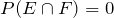. Therefore: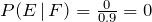.

Example 6.4.6

Given P(F | E) = 0.5, and P() = 0.3. Find P(E).

Solution

Using the conditional probability formula, we get: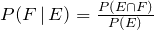Substituting: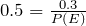or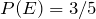Example 6.4.7

In a family of three children, find the conditional probability of having two boys and a girl, given that the family has at least two boys.
Solution

Let event E be that the family has two boys and a girl, and let F be the probability that the family has at least two boys. We want to find P(E | F). We list the sample space along with the events E and F:

S = {BBB , BBG , BGB , BGG , GBG, GBB , GGB , GGG}
E = {BBG , BGB , GBB} and F = {BBB , BBG , BGB , GBB}= {BBG , BGB , GBB}
Therefore,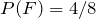, and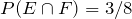.
And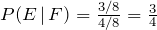.

Example 6.4.8

At a university, 65% of the students use Windows computers, 50% use Apple (Mac) computers, and 20% use both. If a student is chosen at random, find the following probabilities.
a. A student uses a Windows computer given that they use a Mac.
b. A student uses a Mac knowing that they use a Windows computer.
Solution
Let event W be that the student uses a Windows computer, and M the probability that they use a Mac.
a.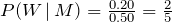b.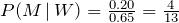.

# Practice questions

1. A die is rolled. Use the conditional probability formula to find the conditional probability that it shows a three if it is known that an odd number has shown.

2. The following table shows the distribution of coffee drinkers by gender:

 Coffee drinker Males (M) Females (F) TOTAL
 Yes (Y) 31 33 64 No (N) 19 17 36 50 50 100

Use the table to determine the following probabilities:

a.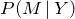b.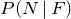c.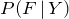3. In the Occupational and Public Health program at Ryerson University, 60% of the students pass Biostatistics, 70% pass Environmental Health Law, and 30% pass both of these courses. If a student is selected at random, find the following conditional probabilities:

a. They pass Biostatistics given that they passed Law

b. They pass Law given that they passed Biostatistics

4. Consider a family of three children. What is the probability of the family having children of both sexes given that the first born child is a boy?

5. If P() = 0.25 and P(F | E) = 0.55, find P(E).

6. A survey of drivers was conducted to determine the number of speeding tickets received among males and females. The data are displayed in the table below.

 Number of tickets Male (M) Female (F) Total 0 425 600 1025 1 250 175 425 2 125 75 200 3 100 50 150 Total 900 900 1800

Use the table to determine the following probabilities:

a. P(0 speeding tickets)

b. P(F | 1 speeding ticket)

c. P(M | at least 2 speeding tickets)